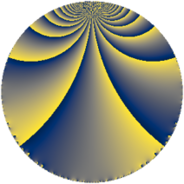# Properties

 Label 143.4.uLevel $143$ Weight $4$ Character orbit 143.u Rep. character $\chi_{143}(4,\cdot)$ Character field $\Q(\zeta_{30})$ Dimension $320$ Newform subspaces $1$ Sturm bound $56$ Trace bound $0$

# Related objects

## Defining parameters

 Level: $$N$$ $$=$$ $$143 = 11 \cdot 13$$ Weight: $$k$$ $$=$$ $$4$$ Character orbit: $$[\chi]$$ $$=$$ 143.u (of order $$30$$ and degree $$8$$) Character conductor: $$\operatorname{cond}(\chi)$$ $$=$$ $$143$$ Character field: $$\Q(\zeta_{30})$$ Newform subspaces: $$1$$ Sturm bound: $$56$$ Trace bound: $$0$$

## Dimensions

The following table gives the dimensions of various subspaces of $$M_{4}(143, [\chi])$$.

Total New Old
Modular forms 352 352 0
Cusp forms 320 320 0
Eisenstein series 32 32 0

## Trace form

 $$320 q - 9 q^{2} - 3 q^{3} - 155 q^{4} - 9 q^{6} - 9 q^{7} + 273 q^{9} + O(q^{10})$$ $$320 q - 9 q^{2} - 3 q^{3} - 155 q^{4} - 9 q^{6} - 9 q^{7} + 273 q^{9} - 256 q^{10} + 150 q^{11} - 60 q^{12} - 114 q^{13} + 20 q^{14} - 63 q^{15} + 661 q^{16} + 155 q^{17} + 195 q^{19} - 333 q^{20} - 693 q^{22} + 92 q^{23} - 1347 q^{24} + 1876 q^{25} - 60 q^{26} + 192 q^{27} + 147 q^{28} - 113 q^{29} - 979 q^{30} + 1032 q^{32} + 306 q^{33} + 41 q^{35} - 1986 q^{36} - 9 q^{37} + 526 q^{38} - 118 q^{39} + 1328 q^{40} + 279 q^{41} + 2273 q^{42} - 568 q^{43} - 654 q^{45} - 657 q^{46} - 1625 q^{48} - 1613 q^{49} - 966 q^{50} - 1496 q^{51} + 3033 q^{52} - 308 q^{53} + 1518 q^{54} + 999 q^{55} + 34 q^{56} + 2175 q^{58} + 2061 q^{59} - 579 q^{61} - 2077 q^{62} - 5754 q^{63} + 9292 q^{64} - 276 q^{65} - 8502 q^{66} - 1272 q^{67} - 4496 q^{68} + 2823 q^{69} - 483 q^{71} - 15123 q^{72} - 2965 q^{74} - 3067 q^{75} + 2142 q^{76} + 3990 q^{77} + 9800 q^{78} + 7460 q^{79} + 4377 q^{80} + 2119 q^{81} - 889 q^{82} + 6840 q^{84} - 5511 q^{85} + 9000 q^{87} + 3727 q^{88} - 6372 q^{89} - 6066 q^{90} - 2018 q^{91} - 5422 q^{92} - 12849 q^{93} + 5449 q^{94} + 3853 q^{95} - 2889 q^{97} + 11394 q^{98} + O(q^{100})$$

## Decomposition of $$S_{4}^{\mathrm{new}}(143, [\chi])$$ into newform subspaces

Label Dim $A$ Field CM Traces $q$-expansion
$a_{2}$ $a_{3}$ $a_{5}$ $a_{7}$
143.4.u.a $320$ $8.437$ None $$-9$$ $$-3$$ $$0$$ $$-9$$